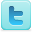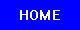Algebra and Calculus
Science Fair Projects
Ideas and Sample Projects by Grade Level

Middle / High School - Grades 7-12
P=Project   E=Experiment

### Algebra

The Algebra and Geometry of Quasicategories [E]
Define unknown mathematical operators [E]
Determine if there is a mathematical solution for the card trick known as The 11th Variation [E]
Investigates the root mean square expectation of the winding number. [E] [E]
Preserving Algebraic Structures on Exact Quasicategories with the K-Theory Functor [E]
What is the effect of putting different variable values in the fractal "Mandel's" equation? [E] [P] [P]
Is Mathematics Discovered or Invented? [E]

### Calculus

Tetrahedral Shoelace Algorithm: Calculating Volume of Irregular Solids [P]
Research the Leibniz and Newton calculus controversy [E]
The Solution of the Dirichlet Problem with Rational Boundary Data [E] [E]
Find out which shape of a fixed perimeter encloses the largest area. [E]
Find out if the trapezoidal approximation or the midpoint approximation gives a more accurate result for the area under the curve. [E] [E]
Is Mathematics Discovered or Invented? [E]
R=Resource
Science Fair Projects Resources [R]
Citation Guides, Style Manuals, Reference [R]

 Mathematics Projects Math Topics Related Subjects Statistics & Probability Geometry & Trigonometry Applied Mathematics Games & Game Theory Number Theory Mathematics Learning Algebra & Calculus Computer Science Intelligence

 Science Fair Project Guide Home Science Fair Project Types The Scientific Method - How to Experiment The Display Board Topics, Ideas, Sample Projects